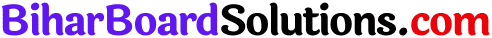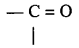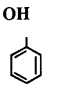# Bihar Board 12th Chemistry VVI Objective Questions Model Set 3 in English

Bihar Board 12th Chemistry Objective Questions and Answers

## Bihar Board 12th Chemistry VVI Objective Questions Model Set 3 in English

Question 1.
Alcohol is formed when one of the hydrogen of Alkane is replaced by
(a) Hydroxyl group
(b) Aldehyde group
(c) Carboxylic group
(d) Chloro group
(a) Hydroxyl group

Question 2.
Group I elements of Periodic Table are known as
(a) Alkali metals
(b) Alkaline earth metals
(c) Inert gases
(d) None
(a) Alkali metalsQuestion 3.
K3[Fe(CN)6] is a
(a) Double slat
(b) Complex compound
(c) Acidic Salt
(d) Common slat
(b) Complex compound

Question 4.
Ammonia changes the moist red litmus paper into
(a) Blue
(b) Green
(c) Black
(d) White
(a) Blue

Question 5.
Polythene is a polymer of
(a) Ethane
(b) Ethene
(c) Propene
(d) Ethyne
(b) EtheneQuestion 6.
The symbol of Helium is
(a) He
(b) Hi
(c) Hm
(d) All
(a) He

Question 7.
The functional group of alcohol is-
(a) —COOH
(b) —CHO
(c)(d) —OH
(d) —OH

Question 8.
H2SO4 is a
(a) Dibasic acid
(b) Monobasic acid
(c) Mono acid-base
(d) Di acid-base
(a) Dibasic acidQuestion 9.
The oxidation number of Fe in K4[Fe(CN)6] is
(a) +2
(b) +3
(c) -2
(d) -3
(a) +2

Question 10.
Buna – N and Buna – S is
(a) Natural rubber
(b) Synthetic rubber
(c) Latex
(d) Polythene
(b) Synthetic rubber

Question 11.
Number of (σ) sigma bonds in CH4 is
(a) 4
(b) 3
(c) 2
(d) 1
(a) 4

Question 12.
Dettol is used as
(a) Disinfectant
(b) Antiseptic
(c) Antimalarial
(d) All
(b) AntisepticQuestion 13.
Copper metal is purified by which of the following method
(a) Electrolytic refining
(b) Zone refining
(c) Chromatography
(d) None
(a) Electrolytic refining

Question 14.
One Faraday electricity is equal to how much Coulombs?
(a) 96550
(b) 96500
(c) 96000
(d) 95500
(b) 96500

Question 15.
The formula of sucrose is
(a) C12H22O12
(b) C12H22O11
(c) C12H24O11
(d) C12H22O10
(b) C12H22O11

Question 16.
Second period of periodic table contains elements
(a) 2
(b) 8
(c) 18
(d) 32
(b) 8Question 17.
Oleum is composed of H2SO4 and which constituent?
(a) SO2
(b) SO3
(c) H2S
(d) HSO4(-)
(b) SO3

Question 18.
Nitrogen & Oxygen are
(a) Metals
(b) Non-metals
(c) Metalloids
(d) None
(b) Non-metals

Question 19.
CH3CH2NH2 is known as
(a) Ethylamine
(b) Propyl amine
(c) Methylamine
(d) Ammonia
(a) Ethylamine

Question 20.
The name of the compoundis
(a) Benzene hydroxide
(b) Phenol
(c) Phenyl
(d) Benzyl Alcohol
(b) PhenolQuestion 21.
White and yellow phosphorous are
(a) Allotropes
(b) Isomers
(c) Isobars
(d) Isotones
(a) Allotropes

Question 22.
Which of the following gas cannot be collected over water?
(a) O2
(b) H2S
(c) SO2
(d) N2
(c) SO2

Question 23.
PCl5 is a
(a) Oxidising agent
(b) Reducing agent
(c) Oxidizing and reducing agent both
(d) None
(a) Oxidising agent

Question 24.
the pH of pure water is
(a) 1
(b) 4
(c) 3
(d) 7
(d) 7Question 25.
Transition elements are also known as
(a) s-block elements
(b) p-block elements
(c) d-block elements
(d) f-block elements
(c) d-block elements

Question 26.
IUPAC name of CH3COOH is
(a) Methanoic acid
(b) Ethanoic Acid
(c) Propanoic acid
(d) Methanol
(b) Ethanoic Acid

Question 27.
The chief ore of Iron is
(a) Magnetite
(b) Siderite
(c) Haematite
(d) All
(d) All

Question 28.
The formula of Nitric acid is
(a) HNO3
(b) H2N2O2
(c) HNO2
(d) HNO4
(a) HNO3Question 29.
The drug used to reduce fever is called
(a) Antipyretic
(b) Analgesic
(c) Antacid
(d) None
(a) Antipyretic

Question 30.
Carbohydrate which yields three to ten monosaccharide units on hydrolysis is called
(a) Monosaccharide
(b) Disaccharide
(c) Oligosaccharide
(d) Polysaccharide
(c) Oligosaccharide

Question 31.
The hybridisation of N in NH3 is
(a) sp3
(b) sp2
(c) sp
(d) d2sp3
(a) sp3Question 32.
A process of heating ore of metal in excess of air below its melting point is called
(a) Roasting
(b) Smelting
(c) Calcination
(d) All
(a) Roasting

Question 33.
Overproduction of acid in stomach can be controlled by
(a) Antacid
(b) Analgesic
(c) Antipyretic
(d) Antibiotic
(a) Antacid

Question 34.
Which of the following gas is mono-atomic?
(a) Chlorine
(b) Helium
(c) Nitrogen
(d) Oxygen